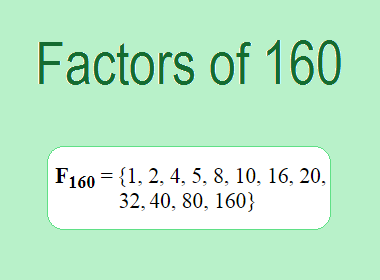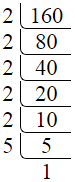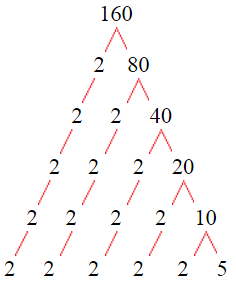# Factors of 160The factors of 160 are 1, 2, 4, 5, 8, 10, 16, 20, 32, 40, 80, and 160 i.e. F160 = {1, 2, 4, 5, 8, 10, 16, 20, 32, 40, 80, 160}. The factors of 160 are all the numbers that can divide 160 without leaving a remainder.

We can check if these numbers are factors of 160 by dividing 160 by each of them. If the result is a whole number, then the number is a factor of 160. Let's do this for each of the numbers listed above:

·        1 is a factor of 160 because 160 divided by 1 is 160.

·        2 is a factor of 160 because 160 divided by 2 is 80.

·        4 is a factor of 160 because 160 divided by 4 is 40.

·        5 is a factor of 160 because 160 divided by 5 is 32.

·        8 is a factor of 160 because 160 divided by 8 is 20.

·        10 is a factor of 160 because 160 divided by 10 is 16.

·        16 is a factor of 160 because 160 divided by 16 is 10.

·        20 is a factor of 160 because 160 divided by 20 is 8.

·        32 is a factor of 160 because 160 divided by 32 is 5.

·        40 is a factor of 160 because 160 divided by 40 is 4.

·        80 is a factor of 160 because 160 divided by 80 is 2.

·        160 is a factor of 160 because 160 divided by 160 is 1.

## How to Find Factors of 160?

1 and the number itself are the factors of every number. So, 1 and 160 are two factors of 160. To find the other factors of 160, we can start by dividing 160 by the numbers between 1 and 160. If we divide 160 by 2, we get a remainder of 0. Therefore, 2 is a factor of 160. If we divide 160 by 3, we get a remainder of 1. Therefore, 3 is not a factor of 160.

Next, we can check if 4 is a factor of 160. If we divide 160 by 4, we get a remainder of 0. Therefore, 4 is also a factor of 160. We can continue this process for all the possible factors of 160.

Through this process, we can find that the factors of 160 are 1, 2, 4, 5, 8, 10, 16, 20, 32, 40, 80, and 160. These are the only numbers that can divide 160 without leaving a remainder.

********************

********************

## Properties of the Factors of 160

The factors of 160 have some interesting properties. One of the properties is that the sum of the factors of 160 is equal to 378. We can see this by adding all the factors of 160 together:

1 + 2 + 4 + 5 + 8 + 10 + 16 + 20 + 32 + 40 + 80 + 160 = 378

Another property of the factors of 160 is that the prime factors of 160 are 2, and 5 only.

## Applications of the Factors of 160

The factors of 160 have several applications in mathematics. One of the applications is in finding the highest common factor (HCF) of two or more numbers. The HCF is the largest factor that two or more numbers have in common. For example, to find the HCF of 160 and 120, we need to find the factors of both numbers and identify the largest factor they have in common. The factors of 160 are 1, 2, 4, 5, 8, 10, 16, 20, 32, 40, 80, and 160. The factors of 120 are 1, 2, 3, 5, 6, 8, 10, 12, 15, 20, 24, 30, 40, 60, and 120. The largest factor that they have in common is 40. Therefore, the HCF of 160 and 120 is 40.

Another application of the factors of 160 is in prime factorization. Prime factorization is the process of expressing a number as the product of its prime factors. The prime factors of 160 are 2, and 5, since these are the only prime numbers that can divide 160 without leaving a remainder. Therefore, we can express 160 as:

160 = 2 × 2 × 2 × 2 × 2 × 5

We can do prime factorization by division and factor tree method also. Here is the prime factorization of 160 by division method,160 = 2 × 2 × 2 × 2 × 2 × 5

Here is the prime factorization of 160 by the factor tree method,160 = 2 × 2 × 2 × 2 × 2 × 5

## Conclusion

The factors of 160 are the numbers that can divide 160 without leaving a remainder. The factors of 160 are 1, 2, 4, 5, 8, 10, 16, 20, 32, 40, 80, and 160. The factors of 160 have some interesting properties, such as having a sum of 378. The factors of 160 have several applications in mathematics, such as finding the highest common factor and prime factorization.